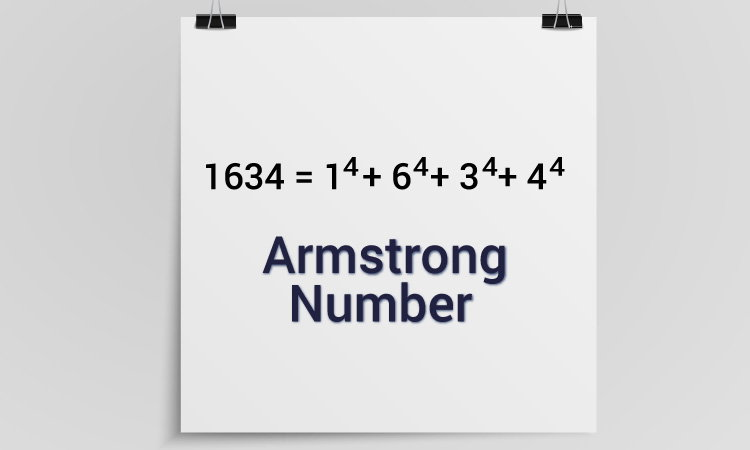# C Program to Check Armstrong Number

Example to check whether an integer (entered by the user) is an Armstrong number or not using while loop and if...else statement.To understand this example, you should have the knowledge of following C programming topics:

A positive integer is called an Armstrong number of order n if

`abcd... = an + bn + cn + dn + ...`

In case of an Armstrong number of 3 digits, the sum of cubes of each digits is equal to the number itself. For example:

```153 = 1*1*1 + 5*5*5 + 3*3*3  // 153 is an Armstrong number.
```

## Example #1: Check Armstrong Number of three digits

``````#include <stdio.h>
int main()
{
int number, originalNumber, remainder, result = 0;

printf("Enter a three digit integer: ");
scanf("%d", &number);

originalNumber = number;

while (originalNumber != 0)
{
remainder = originalNumber%10;
result += remainder*remainder*remainder;
originalNumber /= 10;
}

if(result == number)
printf("%d is an Armstrong number.",number);
else
printf("%d is not an Armstrong number.",number);

return 0;
}``````

Output

```Enter a three digit integer: 371
371 is an Armstrong number.```

## Example #2: Check Armstrong Number of n digits

``````
#include <stdio.h>
#include <math.h>

int main()
{
int number, originalNumber, remainder, result = 0, n = 0 ;

printf("Enter an integer: ");
scanf("%d", &number);

originalNumber = number;

while (originalNumber != 0)
{
originalNumber /= 10;
++n;
}

originalNumber = number;

while (originalNumber != 0)
{
remainder = originalNumber%10;
result += pow(remainder, n);
originalNumber /= 10;
}

if(result == number)
printf("%d is an Armstrong number.", number);
else
printf("%d is not an Armstrong number.", number);

return 0;
}``````

Output

```Enter an integer: 1634
1634 is an Armstrong number.```

In this program, the number of digits of an integer is calculated first and stored in n variable. And, the pow() function is used to compute the power of individual digits in each iteration of the while loop.